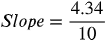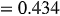1
45
views
17a
Problem

Problem 17a

Chapter 1: Functions and ModelsTextbook ExpertVerified Tutor
21 Oct 2021

Given information

Let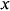denote the depth below the ocean surface and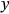denotes the water pressure.

Let us take the coordinates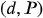Given with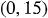as the initial coordinates.

Step-by-step explanation

Step 1.

The relationship is linear, since the growth of water pressure with depth increases at a constant rate.

The slope of a given straight line can be found by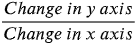.

In this case,